# Class 12 Physics: CBSE Sample Question Paper- Term I (2021-22)- 1 Notes | Study Physics Class 12 - NEET

## NEET: Class 12 Physics: CBSE Sample Question Paper- Term I (2021-22)- 1 Notes | Study Physics Class 12 - NEET

The document Class 12 Physics: CBSE Sample Question Paper- Term I (2021-22)- 1 Notes | Study Physics Class 12 - NEET is a part of the NEET Course Physics Class 12.
All you need of NEET at this link: NEET

Class-XII
Time: 90 Minutes
Max. Marks: 35

General Instructions :

1. The Question Paper contains three sections.
2. Section A has 25 questions. Attempt any 20 questions.
3. Section B has 24 questions. Attempt any 20 questions.
4. Section C has 6 questions. Attempt any 5 questions.
5. All questions carry equal marks.
6. There is no negative marking.
Section - A

This section consists of 25 multiple choice questions with overall choice to attempt any 20 questions. In case more than desirable number of questions are attempted, ONLY first 20 will be considered for evaluation.

Q.1: Which of the following is NOT the property of equipotential surface?
(a) They do not cross each other.
(b) The rate of change of potential with distance on them is zero.
(c) For a uniform electric field, they are concentric spheres.
(d) They can be imaginary spheres.

As all other statements are correct. In uniform electric field, equipotential surfaces are never concentric spheres but are planes ⊥ to electric field lines.

Q.2: Two point charges + 8q and –2q are located at x = 0 and x = L respectively. The point on x axis at which net electric field is zero due to these charges is
(a) 8L

(b) 4L
(c) 2L
(d) L

Let P be the observation point at a distance r from –2q and at (L + r) from + 8q.

Given Now, Net EFI at P = 0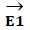= EFI (Electric Field Intensity) at P due to + 8q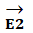= EFI (Electric Field Intensity) at P due to – 2q=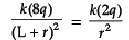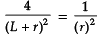4r2 = (L + r)2
2r = L + r
r = L
∴ P is at x = L + L = 2L from origin

∴ Correct option is (C) 2L.

Q.3: An electric dipole of moment p is placed parallel to the uniform electric field. The amount of work done in rotating the dipole by 90° is
(a) 2pE
(b) pE
(c) pE/2
(d) Zero

W = pE (cos θ1 – cos θ2)
θ1 = 0°
θ2= 90°
W = pE (cos 0° – cos 90°)
= pE (1 – 0)
= PE

Q.4: Three capacitors 2 µF, 3 µF and 6 µF are joined in series with each other. The equivalent capacitance is:
(a) 1/2 µF
(b) 1 µF
(c) 2 µF

(d) 11 µF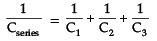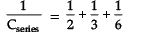3+2+1 / 6 = 6/6

Cseries= 1 µF

Q.5: Two point charges placed in a medium of dielectric constant 5 are at a distance r between them, experience an electrostatic force ‘F’. The electrostatic force between them in vacuum at the same distance r will be:
(a) 5F
(b) F
(c) F/2
(d) F/5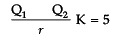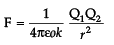Q1________Q2

Force in the charges in the air is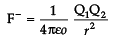.

= KF

= 5F

Q.6: Which statement is true for Gauss law?
(a) All the charges whether inside or outside the gaussian surface contribute to the electric flux.
(b) Electric flux depends upon the geometry of the gaussian surface.
(c) Gauss theorem can be applied to non-uniform electric field.
(d) The electric field over the gaussian surface remains continuous and uniform at every point.

All other statements except (iv) are incorrect.
The electric field over the Gaussian surface remains continuous and uniform at every point.

Q.7: A capacitor plates are charged by a battery with ‘V’ volts. After charging, battery is disconnected and a dielectric slab with dielectric constant ‘K’ is inserted between its plates, the potential across the plates of a capacitor will become:
(a) Zero
(b) V/2
(c) V/K

(d) KV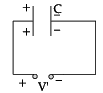Battery is disconnected.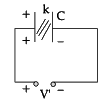Q = Charge remains context C′ = KC
Q′ = C′V′
Q = C′V′
Q = KCV′

V′ =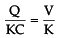Q.8: The best instrument for accurate measurement of EMF of a cell is
(a) Potentiometer
(b) metre bridge
(c) Voltmeter
(d) ammeter and voltmeter

Potentiometer

Q.9: An electric current is passed through a circuit containing two wires of same material, connected in parallel. If the lengths and radii of the wires are in the ratio of 3 : 2 and 2 : 3, then the ratio of the current passing through the wire will be
(a) 2 : 3
(b) 3 : 2
(c) 8 : 27
(d) 27 : 8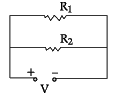I1 : I2 = 3 : 2
r1 : r2 = 2 : 3
I1 : I2 = ?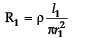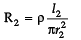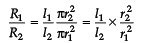=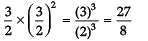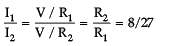Q.10: By increasing the temperature, the specific resistance of a conductor and a semiconductor:
(a) increases for both.
(b) decreases for both.
(c) increases for a conductor and decreases for a semiconductor.
(d) decreases for a conductor and increases for a semiconductor.

Specific resistance of a conductor increases and for a semiconductor decreases with increase in temperature because for a conductor, a temperature:
coefficient of resistivity, a = + ve
and for a semiconductor, a = – ve

Q.11: We use alloys for making standard resistors because they have:
(a) low temperature coefficient of resistivity and high specific resistance.
(b) high temperature coefficient of resistivity and low specific resistance.
(c) low temperature coefficient of resistivity and low specific resistance.
(d) high temperature coefficient of resistivity and high specific resistance.

Alloys have low temperature coefficient of resistivity and high specific resistance. If a = low, the value of ‘R’ with temperature will not change much and specific resistance is high, then required length of the wire will be less.

Q.12: A constant voltage is applied between the two ends of a uniform metallic wire, heat ‘H’ is developed in it. If another wire of the same material, double the radius and twice the length as compared to original wire is used, then the heat developed in it will be:
(A) H/2
(B) H
(C) 2H
(D) 4H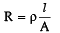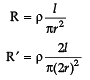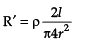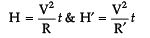∵ V = constant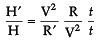=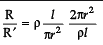H′ /H = 2/1
H′ = 2H

Q.13: If the potential difference V applied across a conductor is increased to 2V with its temperature kept constant, the drift velocity of the free electrons in a conductor will:
(a) remain the same.
(b) become half of its previous value.
(c) be double of its initial value.
(d) become zero.

We know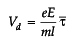=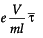If temperature is kept constant, relaxation time τ - will remain constant and e, m are also constants.
Vd ∝ V
Vd ∝ 2V

Q.14: The equivalent resistance between A and B is: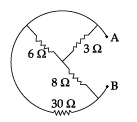(a) 3 ohms
(b) 5.5 ohms
(c) 7.5 ohms
(d) 9.5 ohms

Redrawing the circuit, we get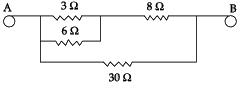3 Ω & 6 Ω are in parallel.
∴ R1 =  3 x 6 / 3 + 6 = 18/9 = 2

Now R1 and 8Ω in series
∴ R2 = R1 + 8 = 2 + 8 = 10 Ω
Now R2 and 30Ωin parallel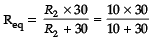= 300/40 = 30/4 = 15 /2
= 7.5 Ω

Q.15: The SI unit of magnetic field intensity is:
(a) AmN–1
(b) NA1m–1
(c) NA2m–2
(d) NA–1m–2

We know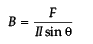SI Unit of B = N/Am = NA1m–1

Q.16: The coil of a moving coil galvanometer is wound over a metal frame in order to:
(a) reduce hysteresis
(b) increase sensitivity
(c) increase moment of inertia
(d) provide electromagnetic damping

The coil of a moving coil galvanometer is wound over metallic frame to provide electromagnetic damping so it becomes dead beat galvanometer.

Q.17: Two wires of the same length are shaped into a square of side 'a' and a circle with radius 'r'. If they carry same current, the ratio of their magnetic moment is:
(a) 2 : π
(b) π : 2
(c) π : 4

(d) 4 : π

l = length of wire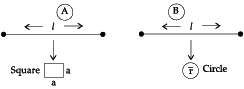Area of a Square = a2
Also here l = 4a
a = l /4
∴ Area = l2 /16
A1 = l2/16
Area of a circle = πr2
Also here, 2πr = l
r = l/2π
Now area =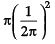A2 = l2/4π
Now Magnetic moment = IA
∴ M1 = IA, & M2 = I A2
Since I (current) is same in both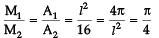M1M2 = π : 4

Q.18: The horizontal component of earth’s magnetic field at a place is 3 times the vertical component. The angle of dip at that place is:
(a) π/6
(b) π/3
(c) π/4
(d) 0

Target law, BV = BH tanδ
tanδ = BV / BH
Given BH = √3BV
tanδ = BV / √3BV = 1/√3
δ = 30° or π / 6 radians.

Q.19: The small angle between magnetic axis and geographic axis at a place is:
(a) Magnetic meridian
(b) Geographic meridian
(c) Magnetic inclination
(d) Magnetic Declination

Correct option is Magnetic declination or Angle of declination. It is the small angle between geographic axis and magnetic axis.

Q.20: Two coils are placed close to each other. The mutual inductance of the pair of coils depends upon the:
(a) rate at which current change in the two coils
(b) relative position and orientation of the coils
(c) rate at which voltage induced across two coils
(d) currents in the two coils

Mutual inductance of a pair of two coils depends on the relative position and orientation of two coils.

Q.21: A conducting square loop of side 'L' and resistance 'R' moves in its plane with the uniform velocity 'v' perpendicular to one of its sides. A magnetic induction 'B' constant in time and space pointing perpendicular and into the plane of the loop exists everywhere as shown in the figure. The current induced in the loop is: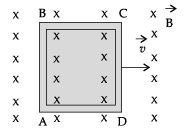(a) BLv/R Clockwise
(b) BLv/R Anticlockwise
(c) 2BLv/R Anticlockwise
(d) Zero

Current induced is I = |e/R|

Now |e| = dφ/dt
But there is no change of flux with time, as B',A' and θ all remain constant with time.
∴ No current is induced.

Q.22: The magnetic flux linked with the coil (in Weber) is given by the equation:

φ = 5t2 + 3t + 16 The induced EMF in the coil at time, t = 4 will be:
(a) - 27 V
(b) - 43 V
(c) - 108 V
(d) 210 V

φ = 5t2 + 3t + 16
|e| = dφ/dt
= d/dt(5t2 + 3t + 16)
= 10t + 3
|e|t=4 = 10(4) + 3 = 43 V
e = - 43 Volts

Q.23: Which of the following graphs represent the variation of current(I) with frequency (f) in an AC circuit containing a pure capacitor?
(a)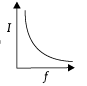(b)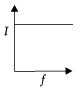(c)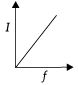(d)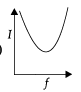I = V / X in pure capacitor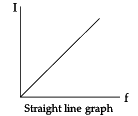=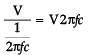Q.24: A 20 volt AC is applied to a circuit consisting of a resistance and a coil with negligible resistance. If the voltage across the resistance is 12 volt, the voltage across the coil is:
(a) 16V
(b) 10V

(c) 8V

(d) 6V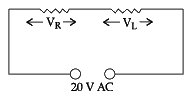VR = Effective voltage across R
∴ VR = Ieff R
VL = Effective voltage across L
VL = Ieff × L
Net V =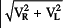=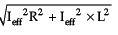20 =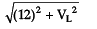(20)= (12)2 + VL2
400 = 144 + VL2
VL = √400 - 144 = √266 = 16 volts

Q.25: The instantaneous values of emf and the current in a series ac circuit are: E = Eo sin ωt and I = Io sin (ωt + π/3) respectively, then it is
(a) Necessarily a RL circuit
(b) Necessarily a RC circuit
(c) Necessarily a LCR circuit
(d) Can be RC or LCR circuit

E = Eo sin ωt
I =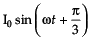as I can lead the voltage in RC and LCR circuit, so it can be RC or LCR circuit.

Section - B
This section consists of 24 multiple choice questions with overall choice to attempt any 20 questions. In case more than desirable number of questions are attempted, ONLY first 20 will be considered for evaluation.

Q.26: Number of charges in 5 coulomb?
(a) electrons 3.125 × 1019
(b) 1
(c) 8.0 × 1019
(d) infinite

According to the quantization of charge, charges of the particle (q) are integral multiple of charge of an electron/proton (e). i.e., q = ne. Therefore, number of elements in 5 coulomb,
n = q /e = 5 / 1.6 x 10-19
= 3.125 x 1019

Q.27: Electric flux emerging out one face of the cube, if a charge Q is placed at the centre of cube, is
(a) Q/ε0
(b) 6Q/ε0
(c) Q/6ε0
(d) Q/4ε
0

Given that a charge Q is placed at the center of the cube, the flux f coming out each through each surface of the cube is same. Since cube has 6 faces, therefore according to Gauss’s law,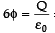⇒ φ = Q/6ε0

Q.28: The electric field in the free space between the sheets is
(a) σ / 2ε0
(b) σ / 4ε0
(c) σ / ε0
(d) zero

Let there are two infinite sheets carry equal and opposite of densities ±σ . At any point, say P, between the sheet, the electric fields are:
E1 =  σ / 2ε0(away from the sheet due to positive charge)
and E2 =  σ / 2ε, (towards the sheet due to negative charge).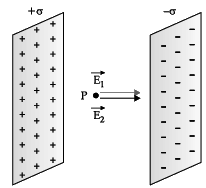Since E1 and Eare in the same direction, the resultant at P due to both sheets is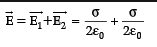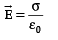Q.29: A parallel plate capacitor is charged to a potential difference V by a DC source and then disconnected. If the distance between the plates is doubled. Capacitance will change to ___________ of initial capacitance.
(a) Half
(b) Double
(c) remain same
(d) four times

Let the initial capacitance = C and distance d. Later capacitance = C’
As capacitance of capacitor is inversely proportional to distance between the plates i.e.
C ∝ 1 / d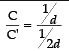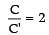⇒ C' = C / 2

Q.30: Work done in moving a 10μC between two points in an equipotential surface is (a) zero
(b) 10 J
(c) 9 kJ
(d) 1 J

The potential difference between two points of equipotential surface is zero,
i.e.,                ΔV = 0
⇒ V2 - V1 = W /q = 0
⇒ W = 0
This indicates that work done in moving a charge in an equipotential surface is zero.

Q.31: Three parallel plate capacitors of capacitance C1 < C2 < C3 are connected in parallel, then its equivalent capacitance of parallel combination Cp is _______ the equivalent capacitance of series combinations.
(a) smaller than
(b) greater than
(c) equal
(d) none of these

If three parallel plate capacitors  are connected in parallel, the potential across these capacitors is same. Let Q1, Q2 and Q3 are the charges on the three capacitors, respectively, such that

Q = Q1+ Q2 + Q3
CpV - C1V + C2v + C3V
Cp — C1 + C2 + C3
Next, if C1, C2 and C3 are connected in series, current flow across them is same, so charge is same. Let V1, V2 and V3  are the potential difference across the three capacitors, respectively, such that
V = V1 + V2 + V3

Q/Cs = Q/C1 + Q/C2 + Q/C3

1/Cs = 1/C1 + 1/C+ 1/C3

This clearly shows that Cs always be less then Cp.

Q.32: An air-filled parallel plate capacitor is charged to a potential difference 90 V and then insulated. A plastic plate is inserted between the plates covering the gap. The potential difference between the plates becomes 15 V. The dielectric constant of plastic is
(a) 2
(b) 3
(c) 6
(d) 8

V= q/C------------------(1)
V = q/C ---------------------(2)
Divide eq. 2 by eq. 1
V/V0 = C0/C
⇒ C0/C = 90/15 = 6

From the definition, it is defined that
C = kC0

⇒ k = 6

Q.33: In an adjoining figure are shown three capacitors C1, Cand C3 joined to a battery. The correct condition will be (Symbols have their usual meanings) :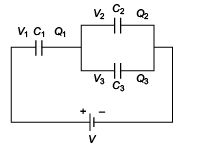(a) Q1=Q2=Q3 and V1=V2=V3=V
(b) Q1=Q2+Q3 and V=V1+V2+V3
(c) Q1=Q2+Q3 and V=V1+V2
(d) Q2=Q3 and V2=V3

It is observed that in series combination charge is same on both the condenser so :       Q1 = Q2 + Q3

Also, the voltages in series will be : V = V1 + V2

Voltages in parallel combination :  V2 = V3

Q.34: The amount of charge Q passed in time t through a cross-section of wire Q = 2t2 + 4t + 4. The value of current at time t = 2 sec is
(a) 8 A

(b) 10 A
(c) 6 A
(d) 12 A

As,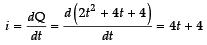At t  = 2 seconds,
i = (4 × 2 ) + 4= 12 A

Q.35: In a meter bridge, the balancing length from the left end is found to be 30 cm. the value of the unknown resistance is
(a) 0.67 W
(b) 0.47 W

(c) 0.27 W
(d) 0.57 W

We know that,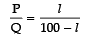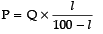As standard resistance of 1W is in the right gap

⇒ P = 1 x 40/60 = 0.67Ω

Q.36: If an unaccelerated charged particle enters in a region having electric field and magnetic field
(a)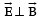(b)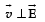(c)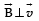(d) None of these

A charged particle enters in a region in an unaccelerated manner if magnetic force and electric field acting on it cancel each other. Therefore,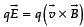, it indicates that E' must be perpendicular to B'. Additionally, it is not necessary that v is perpendicular to E' or E' perpendicular to vB' .

Q.37: An electron moving with the velocity of 106 m/s enters in a magnetic field and follow the circular path having radius of 0.1 m, then the intensity of magnetic field must be
(a) 4.7 × 10–5 T
(b) 5.7 × 10–5 T
(c) 6.7 × 10–5 T
(d) 7.7 × 10–5 T

Given that v = 106 m/s, R = 0.10 m/s me = 9.1 × 10–31 kg, q = 1.6 × 10–19C
As we know that,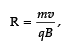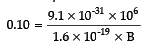⇒ B =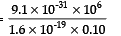⇒ B = 5.7 × 10–5 T

Q.38: A cell is connected between the two points of a circular conductor so that I1 and I2 currents are flowing in two parts of the circular conductor of radius a. The magnetic field at the center of the loop is:
(a)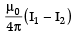(b)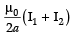(c)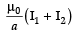(d) zero

Let land l2 be the length two part of the conductor and r be the resistance per unit length of the conductor. Therefore, the resistance of first portion and second portion i.e., R1 = l1ρ and R2 = l2ρ . Since, potential difference across the end equal, so,
I1R1 = I2R2

or I1l1r = I2l2r ...(1)
I1l1 = I2l2/

The magnetic field at the center of the loop,
= B1 - B2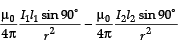= 0

Q.39: A bar magnet having magnetic moment 0.6 JT–1 is placed in a uniform magnetic field of 0.15 T. The potential energy of a magnet, when it is in stable equilibrium
(a) zero
(b) – 0.96 J
(C) -0.096 J
(D) – 9.60 J

In stable equilibrium, Potential energy U is defined as U = – MB

= – (0.6) (0.6)

= – 0.096 J

Q.40: A magnet of magnetic moment 10 C.G.S. is freely suspended in a uniform magnetic field of intensity of 0.3 units. The amount of work done in deflecting it with an angle of 60° in C.G.S. unit is
(a) 2.5
(b) 5.5
(c) 1.5
(d) 3.5

work done is defined as,
W = -MB (1 - cosθ )
= 10 x 0.3 (1 cos60o)
= 3/2
= 1.5

Q.41: If relative permittivity of a medium is 2000. The absolute permeability in S.I. unit is
(a) 8π × 10–4
(b) 2π × 10–4
(c) 4π × 10–4
(d) π × 10–4

As, μ = μ0μr
= (4π x 10-7) x 2000
= 8π x 10-4

Q.42: A magnetic field of 5 × 103 amp/m produces a magnetic flux density of 2π Tesla. The relative permeability is
(a) 10–3
(b) 103
(c) 104
(d) 10
–4

Given that magnetic field intensity = 5 × 103A/m and, magnetic flux density= 2π Tesla the relative permeability, i.e.,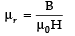=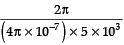= 103

Q.43: Two coils have a mutual inductance of 0.002 H. The current changes in a first coil according to I = I0sin ωt where, I0 = 5A and ω = 20π radian/sec. The maximum value of e.m.f in second coil is
(a) π
(b) 0.1π
(c) 0.2π
(d) 0.3π

E.m.f is defined as:
ε = dl/dt
= 0.002 × I0cosωt × ω
⇒ εmax = 0.002 x I0 x ω

⇒ = 0.002 x 5 x 20π

⇒ = 0.200π

Q.44: An AC generator of 220 V having internal resistance of 5 Ω and external resistance of 105 Ω. What is the power developed in the external resistance?
(a) 420 W
(b) 400 W
(c) 520 W
(d) 500 W

Total resistance = internal resistance + external resistance = 110W.

Therefore, current developed
= 220/110 = 2 A

Power developed in the external resistance = I2Rext
= 4 × 105 = 420 W

Given below are two statements labelled as Assertion (A) and Reason (R)
Directions: In the following questions, a statement of Assertion (A) and is followed by a statement of Reason (R). Mark the correct choice as:
(a) Both Assertion (A) and Reason (R) are true, and Reason(R) is the correct explanation of (A).
(b)
Both Assertion (A) and Reason (R) are true, but Reason (R) is not the correct explanation of Assertion (A).
(c)
Assertion (A) is true, but Reason (R) is false.
(d)
Assertion (A) is false, but Reason (R) is true.
Q.45:
Assertion (A): Coulomb force on any charged particle is the vector sum of the forces experienced on that charge due to other charges.
Reason (R):  Coulomb force between two charges depends on the magnitude of two-point charges and the distance between them

Coulomb force on a charged particle is the vector sum of forces experienced on that charge due to other charges. It is the principle of superposition. Hence, assertion is  true.

Coulomb’s law states that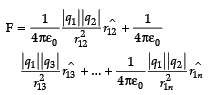Therefore, Reason is true.

Q.46:
Assertion (A): When conducting slab is completely filled between the space of parallel plate capacitors, its capacitance becomes infinite.
Reason (R): Electric field inside a conductor is zero resulting in a zero potential difference between the plates.

When a conducting slab is completely filled between the plates of the capacitor, its capacitance changes accordingly Cmedium = KCair , where Cmedium = Capacitance of a capacitor on loading a medium between the plates and Cair = capacitance of parallel plate capacitor. Since the dielectric constant K of a conductor is infinity. Therefore Cmedium = ∞. Assertion is true. If an electric field is zero, K becomes infinite. Therefore, it is a correct explanation of assertion.

Q.47:
Assertion (A): Voltmeter measure the emf of a cell when it is connected across the terminal of cell.
Reason (R): Terminal potential is given by V = E – ir.

Since, the resistance of voltmeter is infinite, no current flows through the circuit when a voltmeter is connected across the terminal of the cell. Hence, voltmeter gives the reading of the emf of the cell. Assertion is true. Emf of a cell is defined as the work done by a cell in forcing per coulomb of charge to flow in circuit. If internal resistance of cell is r, there is some potential drop across the cell, which is ir. When a cell is connected through the resistance R and applied terminal potential is V, emf of a cell is

E = i(R + r))

⇒ E = iR + ir

⇒ E = V = ir

⇒ V = E - ir
Where, V is terminal potential across the terminals. Therefore, reason is true. So, both assertion and reason are true but reason is not the correct explanation of resistance.

Q.48:
Assertion (A): A compass needle when placed at Earth’s magnetic pole rotates in vertical plane.
Reason (R): The Earth has only vertical component of its magnetic field at the poles.

Magnetic needle can rotate in horizontal plane only. But at poles, there is no horizontal component of Earth’s magnetic field.
So, the needle will remain horizontal and will point in any direction. Hence the assertion is false. At poles, Earth has only vertical components of its magnetic field. Hence, the reason is true.

Q.49:
Assertion (A): At resonance, the current becomes minimum in a series LCR circuit.
Reason (R): At resonance, voltage and current are in same phase in a series LCR circuit.

At resonance, XL = XC' so the circuit impedance becomes minimum and resistive and hence the current becomes maximum. So, the assertion is false. At resonance, XL = XC' so the circuit impedance becomes resistive. In resistive circuit voltage and current are alway

Section - C
This section consists of 6 multiple choice questions with an overall choice to attempt any 5. In case more than desirable number of questions are attempted, ONLY first 5 will be considered for evaluation.

Q.50: Five charges, q each are placed at the corners of a regular pentagon of side ‘a’. Electric field at the centre of the pentagon is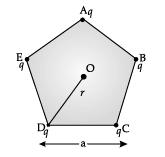(a) q/4πε0r2
(b) 5q/4πε0r2
(c) 0
(d) 5q/4πε0a
2

The point O is equidistant from all the charges at the end point of pentagon. So, due to symmetry, the forces due to all the charges are cancel out each other. So, electric field at the centre is zero.

Q.51: Figure shows the electric field lines around three-point charges A, B and C.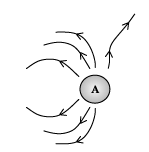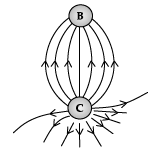(a) A and B are positive, C is negative
(b) A and C are positive, B is negative
(c) B is positive, A and C are negative
(d) A is positive, B and C are negative.

Electric lines of forces diverge out from positive charge. So, A and C are positive charges and B is negative charge.

CASE STUDY

Read the following text and answer the following questions on the basis of the same:
At power plant, a transformer increases the voltage of generated power by thousands of volts so that it can be sent of long distances through high-voltage transmission power lines. Transmission lines are bundles of wires that carry electric power from power plants to distant substations.
At substations, transformers lower the voltage of incoming power to make it acceptable for highvolume delivery to nearby end-users.
Electricity is sent at extremely high voltage because it limits so-called line losses. Very good conductors of electricity also offer some resistance and this resistance becomes considerable over long distances causing considerable loss.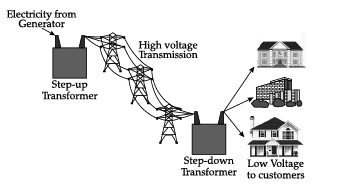At generating station, normally voltage is stepped up to around thousands of volts. Power losses increase with the square of current. Therefore, keeping voltage high current becomes low and the loss is minimized.
Another option of minimizing loss is the use of wires of super-conducting material. Super-conducting materials are capable of conducting without resistance, they must be kept extremely cold, nearly absolute zero, and this requirement makes standard super-conducting materials impractical to use. However, recent advances in super-conducting materials have decreased cooling requirement. In Germany recently 1 km super-conducting cable have been installed connecting the generating station and the destination. It has eliminated the line loss and the cable is capable of sending five times more electricity than conventional cable. Using super-conducting cables Germany has also get rid of the need of costly transformers.
Transformers generate waste heat when they are in operation and oil is the coolant of choice. It transfers the heat through convection to the transformer housing, which has cooling fins or radiators similar to heat exchangers on the outside.
Flush point is a very important parameter of transformer oil. Flashpoint of an oil is the temperature at which the oil ignites spontaneously. This must be as high as possible (not less than 160° C from the point of safety).
Fire point is the temperature at which the oil flashes and continuously burns. This must be very high for the chosen oil (not less than 200° C).
Q.52: Which of the following statement is true for long distance transmission of electricity?
(a) Step-down transformer is used at generating station and step-up transformer is used at destination substation.
(b) Step-down transformers are used at generating station and destination substation.
(c) Step-up transformers are used at generating station and destination substation.
(d) None of the above

At power plant, a step-up transformer increases the voltage of generated power by thousands of volts, so that it can be sent of long distances through high-voltage transmission power lines.
At substations, step-down transformers lower the voltage of incoming power to make it acceptable for high-volume delivery to nearby end-users.

Q.53: Super-conducting transmission line has the following advantages:
(a) Resistance being zero, there is no I2R loss.
(b) There is no requirement of costly step-up and step-down transformers.
(c) Cable is capable of sending more electricity.
(d) All of the above

Super-conducting materials are capable of conducting without resistance. So, this eliminates the line loss and the cable is capable of sending more electricity than conventional cable. Using super-conducting cables, one can get rid of the need of costly transformers.

Q.54: Why does stepping up voltages reduce power loss?
(a) Since resistance of conductor decreases with increase of voltage
(b) Since current decreases with increase of voltage
(c) Both of the above
(d) None of the above

At generating station, normally voltage is stepped up to around thousands of volts. Power losses increase with the square of current. Therefore, keeping voltage high, current becomes low and the loss is minimized.

Q.55: Oil transfers heat from transformer winding by the process of:
(a) convection
(b) conduction
(d) All of these

Transformers generate waste heat when they are in operation and oil is the coolant of choice. It transfers the heat through convection to the transformer housing.

The document Class 12 Physics: CBSE Sample Question Paper- Term I (2021-22)- 1 Notes | Study Physics Class 12 - NEET is a part of the NEET Course Physics Class 12.
All you need of NEET at this link: NEETUse Code STAYHOME200 and get INR 200 additional OFF

## Physics Class 12

134 videos|388 docs|213 tests

### How to Prepare for NEET

Read our guide to prepare for NEET which is created by Toppers & the best Teachers

Track your progress, build streaks, highlight & save important lessons and more!

,

,

,

,

,

,

,

,

,

,

,

,

,

,

,

,

,

,

,

,

,

;